# Community

## FlexiLayout Studio CharacterString Regex

Hello, I have a problem that I can't solve. A number that is divided into 2 lines, I find it with the activity Paragraph, but after a while it turned out that the documents were of poor quality and there were unnecessary characters in the numbers or the numbers did not match what was written and I switched to Character String and I want to use Regex to strictly write the number format, and my problem is that I can't move to a new line using regex.Wrote like this [A-Z]{1}N{3}[A-Z]{2}N{2}\r\n[A-Z]{2}N{6} did without \r but still can't find the number.
I would like to do it the way I want, but if I'm wrong, correct me, since I'm new in this field and just learning, don't judge me strictly.My goal is to find a number that matches the correct format in this case X111XX11/XX111111 and if it does not match this format, then it should not be found.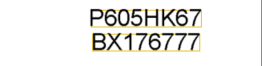0 out of 0 found this helpful

•I don't think that FlexiCapture's RegEx engine recognizes carriage returns and line feeds. (\r\n). Not sure how you would use something like .Net RegEx in FLS.

Keep in mind that you can always have FLS find the data, but then use rules in the document definition to verify that data. In the document definition (or a stage script in the workflow) you can more easily reference .Net and use a more common RegEx engine.

If FLS finds data that you do not want, remove it in the project, in a rule or a stage script.

RegEx Script

Extended Regular Expressions

•I achieved that I created a rule and gave out the formats of the car number there, but the problem is that if the text contains other characters, then the value is false, and I want to ensure that if the context contains characters, then from this context it will get the car number that corresponds to the regular expression

using System.Text.RegularExpressions;
using System;

string pattern = @"^[A-ZА-Я]{2}\d{4}[A-ZА-Я]{2}/[A-ZА-Я]{2}\d{4}[A-ZА-Я]{2}\$|^\d{2}[A-ZА-Я]{2}\d{3}/\d{2}[A-ZА-Я]{2}\d{3}\$|^[A-ZА-Я]{2}\d{5}/[A-ZА-Я]{2}\d{5}\$|^\d{3}[A-ZА-Я]{2}\d{2}/\d{3}[A-ZА-Я]{1}\d{2}\$|^[A-ZА-Я]{2}\d{3}[A-ZА-Я]{2}/[A-ZА-Я]{2}\d{3}[A-ZА-Я]{1}\$|^[A-ZА-Я]{1}\d{3}[A-ZА-Я]{2}\d{2}/[A-ZА-Я]{2}\d{6}\$|^[A-ZА-Я]{2}\d{4}-\d{1}/[A-ZА-Я]{1}\d{4}[A-ZА-Я]{1}-\d{1}\$|^\d{3}[A-ZА-Я]{2}\d{2}/\d{2}[A-ZА-Я]{3}\d{2}\$";

Context.Field("car_number").Text = Context.Field("car_number").Text.Replace("\r\n", "/").Replace("\r", "/").Replace("\n", "/").Replace(" ","");

Regex rgx = new Regex(pattern, RegexOptions.IgnoreCase );
Match match = rgx.Match(Context.Field("car_number").Text);

if( match.Success ) {
Context.Field( "car_number" ).Text=Context.Field( "car_number" ).Text;
}else{
Context.Field( "car_number" ).Text="";
}

the script outputs like this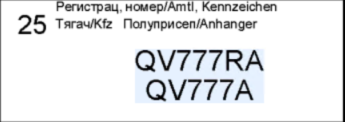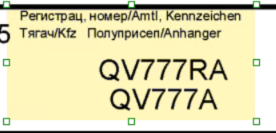here are 2 results when everything is fine and without extra characters, everything works and when the ratio is a little off, I want to do it so that everything is discarded from this area and I would get the car number.if the script is written crooked, then do not judge strictly, I'm just learning thanks for the help

•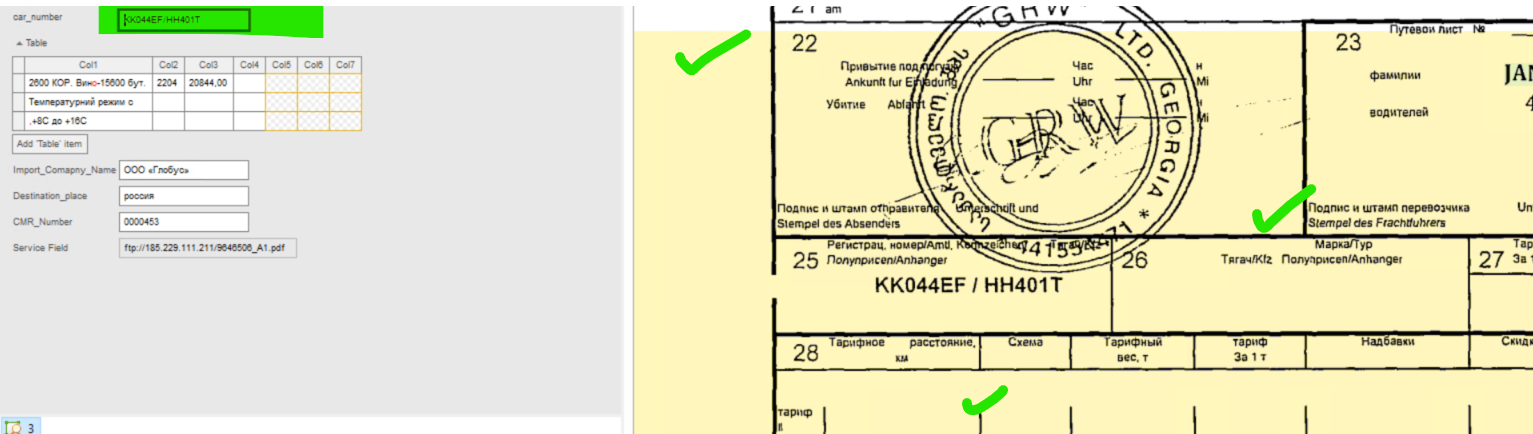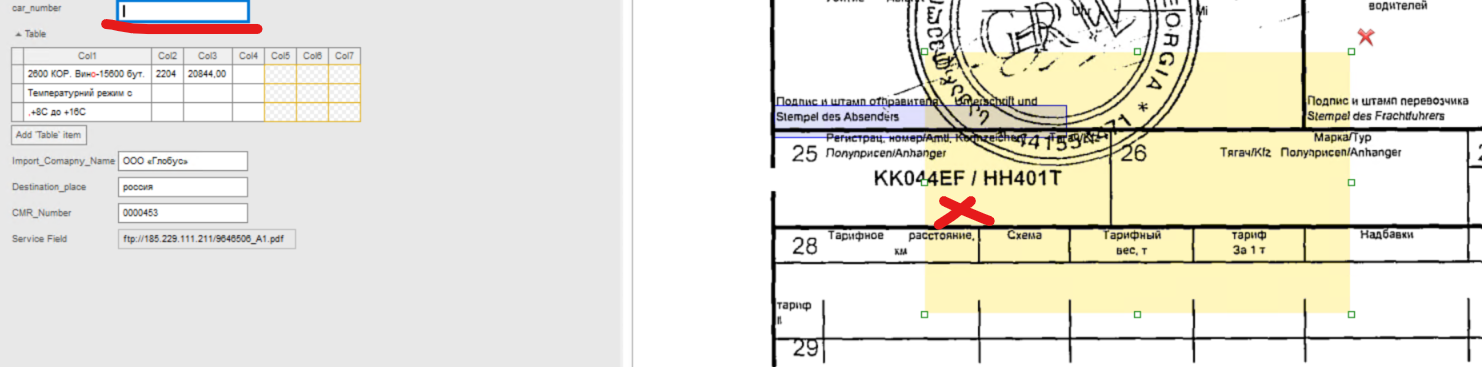•